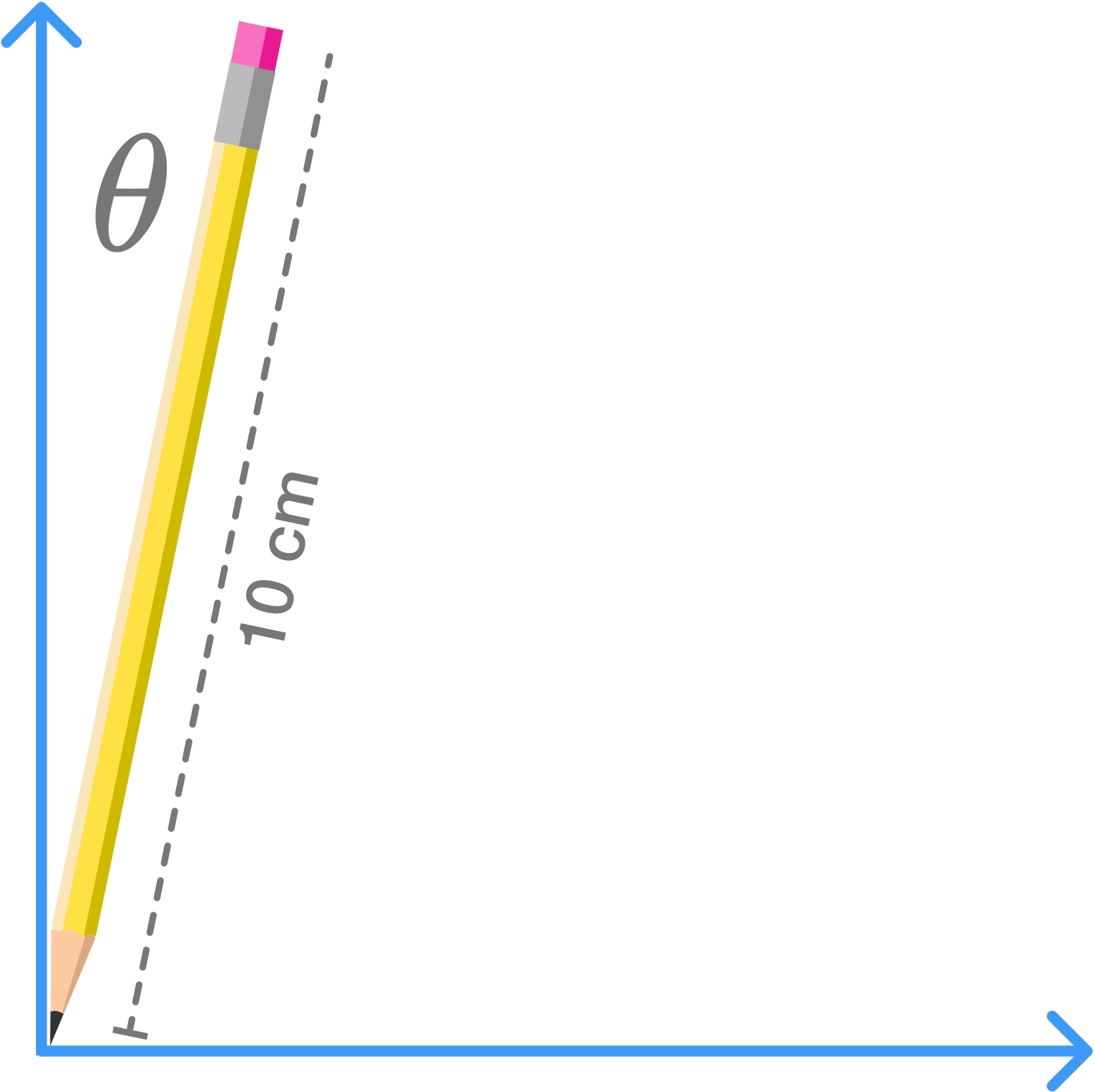# Falling PencilEstimate the time it takes for a pencil of length $l=\si{10\ \centi\meter}$ to fall if the pencil starts from rest at the angle $\theta_0=\si{0.001\ \radian}$ with the vertical.

• Take the gravitational acceleration to be $g=\SI{10}{\meter\per\second\squared}$.